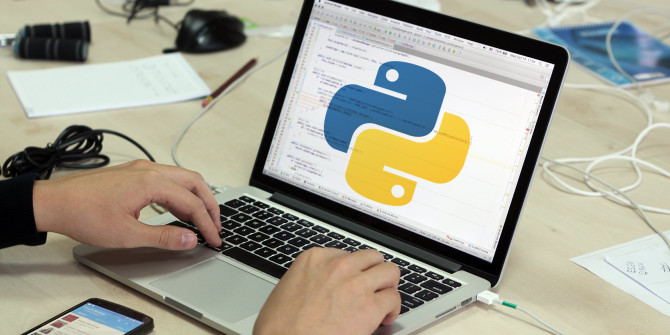Top 10 Programming Languages to Learn in 2019Page Contents

## Use Cases

1. Printing with variables
2. Beautifying the printed statements
3. Both of them

## Floating Point Numbers

Floating point numbers can use format %m.nf where m represents total minimum number of digits a string should contain. n represents the number of digits after the decimal point.

Extra spaces may also be filled with white-spaces if entire number doesn’t have many digits

```>>> print("%3.4f" % 1234.33)
1234.3300
>>> print("%3.1f" % 1234.33)
1234.3```

### Explanation

The format %3.4f specified that the printed number should contain 4 digits after the decimal hence two zeroes were added at the last. Similarly, the format %3.1f specified that the printed number should contain only 1 digit and therefore the second ‘3’ after the decimal was truncated.

## String

You can use %s to format string into your print statement

```>>> string1= 'world'
>>> print('Hello %s' %string1)
Hello world
>>> ```

## Conversion Format Method

The format methods %s and %r converts any python objects into a string using two separate methods set() and repr(). Almost any python object can be printed using this technique. Let’s see few examples:

```>>> print('Number - %s, String - %s, List - %s' %(num, string, list1))
Number - 1.3, String - Hello, List - ['a', 'b']
>>> print('Number - %r, String - %r, List - %r' %(num, string, list1))
Number - '1.3', String - 'Hello', List - ['a', 'b']```

## Multiple Formatting

We can also pas a tuple to the modulo symbol to place multiple formats in your print statements:

```>>> print("string - %s , float - %3.5f" % ('hello world',3.144343))
string - hello world , float - 3.14434```

## String.format() method

This is one of the best way to format strings. The syntax is:

```>>> print("string - {0} , float - {1}".format('hello world',3.144343))
string - hello world , float - 3.144343
>>> print("string - {1} , float - {0}".format(3.144343,'hello world'))
string - hello world , float - 3.144343
>>>>>> print("{0}{0}{0}".format("hello "))
hello hello hello ```

It is worth to note here that we can index the arguments passed in format() method.

It is also extremely helpful in cases where the same object has to be printed again and again. We can also pass the objects as keyword agruments:

```>>> print("Hello {w}".format(w="world"))
Hello world```